# The linear combination of two polygonal numbers is a perfect square

Mei Jiang and Yangcheng Li
Notes on Number Theory and Discrete Mathematics
Print ISSN 1310–5132, Online ISSN 2367–8275
Volume 26, 2020, Number 2, Pages 105—115
DOI: 10.7546/nntdm.2020.26.2.105-115

## Details

### Authors and affiliations

Mei JiangSchool of Mathematics and Statistics, Changsha University of Science and Technology,
Hunan Provincial Key Laboratory of Mathematical Modeling and Analysis in Engineering,
Changsha, 410114, China

Yangcheng LiSchool of Mathematics and Statistics, Changsha University of Science and Technology,
Hunan Provincial Key Laboratory of Mathematical Modeling and Analysis in Engineering,
Changsha, 410114, China

### Abstract

By the theory of Pell equation and congruence, we study the problem about the linear combination of two polygonal numbers is a perfect square. Let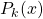denote the-th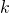-gonal number. We show that if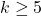,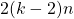is not a perfect square, and there is a positive integer solutionofsatisfyingthen the Diophantine equation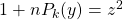has infinitely many positive integer solutions. Moreover, we give conditions aboutsuch that the Diophantine equation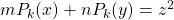has infinitely many positive integer solutions.

### Keywords

• Polygonal number
• Diophantine equation
• Pell equation
• Positive integer solution

• 11D09
• 11D72

### References

1. Bencze, M. (2012). Proposed Problem 7508, Octogon Mathematical Magazine, 13 (1B), 678.
2. Chen, J. P. (2012). The squares with the form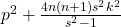, Natural Science Journal of China West Normal University, 33 (2), 196–198, 217.
3. Cohen, H. (2007). Number Theory, Vol. I: Tools and Diophantine Equations, Graduate Texts in Mathematics, Springer.
4. Deza E., & Deza M. M. (2012). Figurate Numbers, Word Scientific.
5. Dickson, L. E. (1934). History of the Theory of Numbers, Vol. II: Diophantine Analysis, Dover Publications.
6. Eggan, L. C., Eggan, P. C., & Selfridge, J. L. (1982). Polygonal products of polygonal numbers and the Pell equation, Fibonacci Quart., 20 (1), 24–28.
7. Guan, X. G. (2011). The squares with the form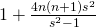, Natural Science Journal of Ningxia Teachers University, 32 (3), 97–107.
8. Guy, R. K. (2007). Unsolved Problems in Number Theory, Springer-Verlag.
9. Hu, M. J. (2013). The positive integer solutions of the Diophantine equation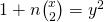,
Journal of Zhejiang International Studies University, 4, 70–76.
10. Ke, S., & Sun, Q. (1980). About Indeterminate Equation, Harbin Institute of Technology Press.
11. Le, M. H. (2007). The squares with the form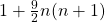, Natural Science Journal of Hainan University, 25 (1), 13–14.
12. Peng, J. Y. (2019). The linear combination of two triangular numbers is a perfect square, Notes on Number Theory and Discrete Mathematics, 25 (3), 1–12.
13. Ran, Y. X., Yan, S. J., Ran, Y. P., & Yang, X. Y. (2008). The squares with the form, Journal of Tianshui Normal University, 28 (5), 9–15.
14. Sun, Z. H. (2009). On the number of representations of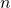by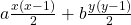, Journal of Number Theory, 129 (5), 971–989.
15. Wu, H. M. (2011). The square numbers with the form, Journal of Zhanjiang Normal College, 32 (3), 20–22.

## Cite this paper

Jiang, M., & Li, Y. (2020). The linear combination of two polygonal numbers is a perfect square. Notes on Number Theory and Discrete Mathematics, 26 (2), 105-115, doi: 10.7546/nntdm.2020.26.2.105-115.